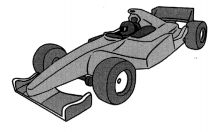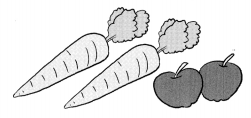# Math in Focus Grade 5 Chapter 9 Practice 7 Answer Key Real-World Problems: Decimals

Practice the problems of Math in Focus Grade 5 Workbook Answer Key Chapter 9 Practice 7 Real-World Problems: Decimals to score better marks in the exam.

## Math in Focus Grade 5 Chapter 9 Practice 7 Answer Key Real-World Problems: Decimals

Question 1.
Mrs. Lee uses 0.025 kilogram of wax to make a candle. On Monday, she made 50 candles. On Tuesday, she made 4 times as many candles as on Monday. How much wax did she use to make the candles on Tuesday?
Explanation:
given information,
on Monday 0.025 km of wax use to make 50 candles.
on Tuesday x kg of wax used to make 4 x 50 = 200 candles.
how much wax ‘x ‘ is to be find out.
0.025 ÷ 50 = 0.0005
0.025 ÷ 50
=0.0005 kilograms of wax is used for one candle.
for 4 x 50 = 200 candles.
0.0005 x 200 = 0.1 kg of wax is used.

Question 2.
One lap of a race track measures 4.68 kilometers. During a race of 56 laps, a driver stops to refuel after completing 48 laps. How many more kilometers does he have to drive to finish the race?37.2 kilometers
Explanation:
one lap = 4.65 kilometers
56 x 4.65 = 260.4 kilometers
48 x 4.65 = 223.2 kilometers
37.2 more kilometers does he have to drive to finish the race.
260.4 – 223.2 = 37.2 kilometers

Question 3.
Mrs. Rahlee bought 300 yards of ribbon to make flowers. She used 1.22 yards to make one large flower. She made 200 such large flowers She used all of the remaining ribbon to make 100 small flowers. What was the length of ribbon Mrs. Rahlee used to make one small flower?
0.56 yards length of ribbon Mrs. Rahlee used to make one small flower.
Explanation:
total 300 yards of ribbon to make flowers.
1.22 yards for one flower.
200 x 1.22 = 244 yards used to make one large flower.
300 – 244 = 56 yards
56 ÷ 100 = 0.56
0.56 yards length of ribbon Mrs. Rahlee used to make one small flower.

Question 4.
Britta bought some carrots and apples for $24.80. A carrot and an apple cost$0.90 altogether. She bought more carrots than apples. The cost of the extra number of carrots was $6.80. How many apples did Britta buy?Answer: 20 apples Explanation: c carrot a apple x is number of carrots y is number of apples c + a = 0.90 x c + y a = 24.80 6.80 + y a = 24.80 y a = 24.80 – 6.8 = 18 But a+c=.90 y=18÷ 0.9 = 20 y is the number of apples Solve. Show your work. Question 5. A plastic tub has a capacity of 1 3.5 quarts. It can hold 3 times as much liquid as a pail. The pail can hold twice as much liquid as a can. Find the capacity of the pail and that of the can in quarts. Answer: 2.25 quarts Explanation: capacity of tub = 13.5 quarts. Tub can hold three times as much as a pail. capacity of pail = 13.5 ÷ 3 = 4.5 quarts. The pail can hold twice as much liquid as a can. capacity of can =4.5 ÷ 2 = 2.25 quarts. Question 6. Marcy paid$35 for 10 kilograms of raisins. She divided the raisins equally into two containers. Then she sold the raisins in the first container at $4.50 per kilogram and those in the second container at$5.50 per kilogram. How much money did Marcy earn after selling all the raisins?
Answer: $15 Explanation: Since she divided raisins equally both containers have 5kg each. Total earnings from selling container one = 4.5 * 5 =$22.5
Total earnings from selling container two = 5.50 * 5 = $27.5 Total earnings from both containers = 22.5 + 27.5 =$50
Total cost of raisins = $35 Total Profit =$50 – $35 =$15